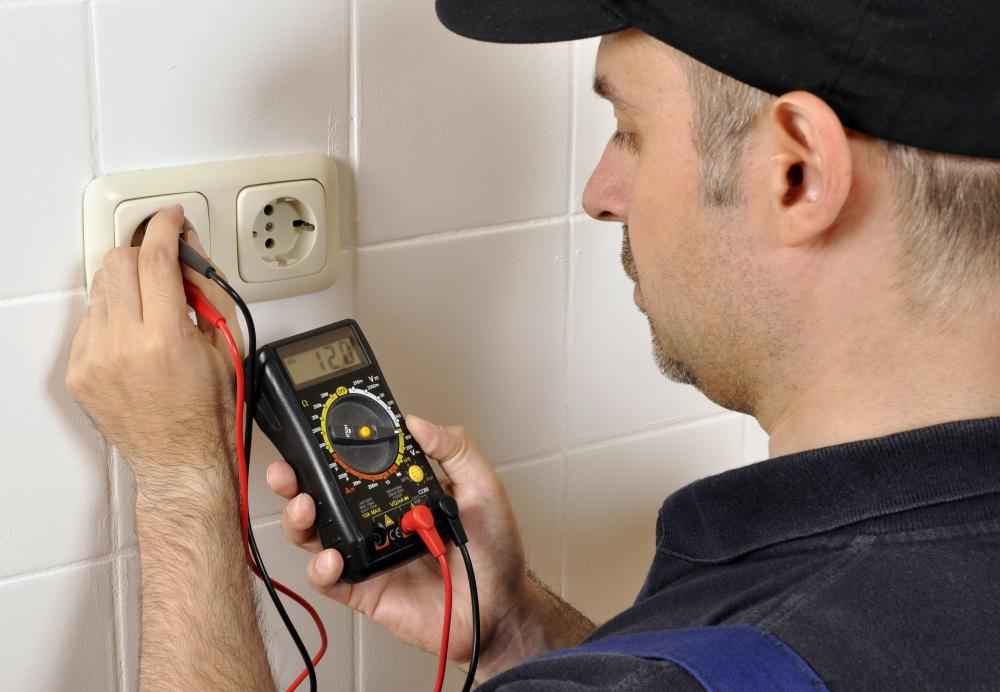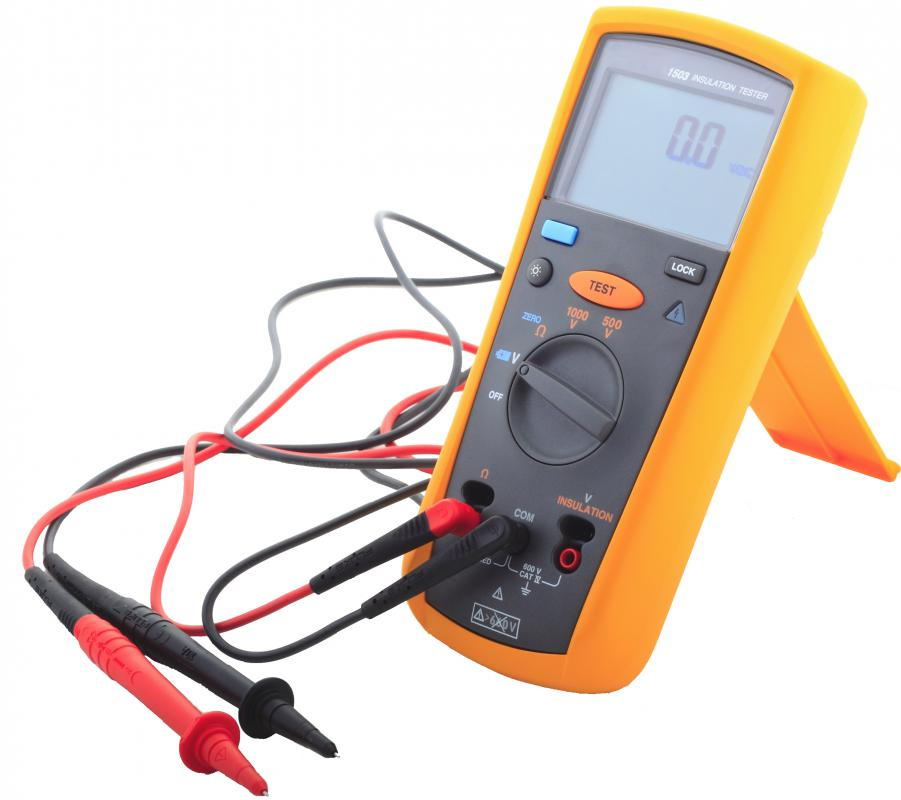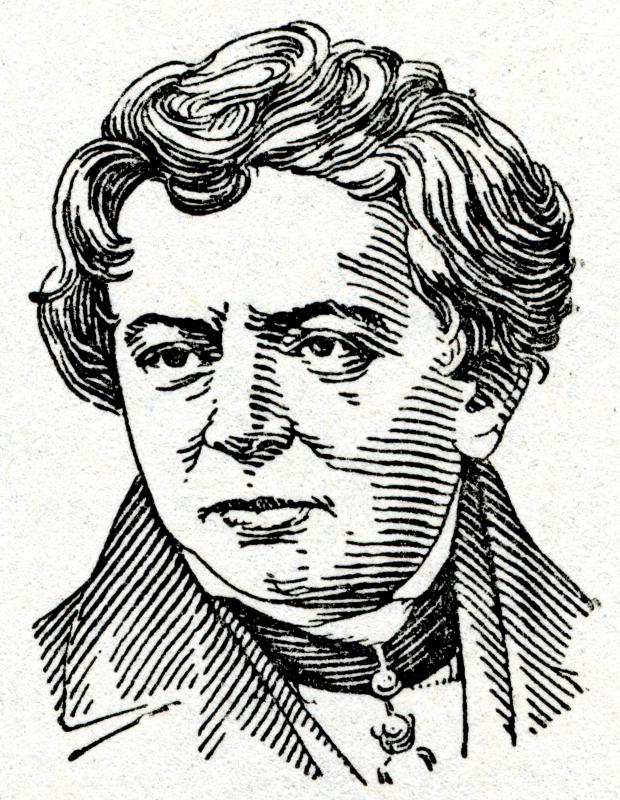# What is a Voltmeter?

Damir Wallener

A voltmeter is a device used to measure the voltage potential between two points in an electrical circuit. First created in the early 1800s, these devices were originally called galvanometers. Technically, they are all ammeters, as they measure current rather than voltage. Although current is measured in amps, Ohm's Law, which establishes the relationship between voltage, current and resistance, can be used to scale the amps to volts.Voltmeters and multimeters are used to measure voltage levels for an outlet or circuit.

Voltmeters were made possible by an 1819 discovery by Hans Oersted. When he passed a current through a wire near a compass needle, he noticed the needle would change direction. The earliest attempts to take advantage of this effect were little more than a coil of wire wrapped around a compass; the stronger the current passing through the wire, the greater the deflection of the compass needle. While functional, these early models were not very accurate.Digital voltmeters have a display, amp controller and sometimes require calibration.

In the late 19th century, Arsene D'Arsonval discovered the instrument could be made much more responsive if the coil around the compass was made smaller, attached to the base of the needle, and surrounded by a circular magnet. This became known as the D'Arsonval movement, and is still used today in analog meters.

Because all measurable electrical properties are related to each other through Ohm's Law, in practice, most voltmeters are actually multimeters capable of measuring voltage, current and resistance. When measuring voltage, a large resistance is placed in line with the coil of the D'Arsonval movement to minimize interference with the circuit being measured. This is also due to Ohm's Law, which states voltage is directly proportional to resistance. By minimizing the voltage consumed by the meter itself, a more accurate measurement of the circuit's voltage can be obtained. Current is inversely proportional to resistance; conversely, to minimize the amount of current flowing into an ammeter, a large resistance is placed in parallel with the coil.

Modern multimeters come with digital displays and may even use digital measuring techniques rather than a D'Arsonval movement. By incorporating microprocessors, they are also capable of advanced functions such as measuring frequency, counting electrical events, and measuring capacitance.Ohm's Law was named for and first described by, German physicist Goerg Ohm.

## You might also Likeanon294422

Are voltmeters even safe at all?

anon239606

How can I measure voltage without using a voltmeter or multimeter at all?

anon47388

to anon26539: Connect a resistor of slightly less than 1kohm in series with this coil. attach the whole system in parallel with the voltage source. read the ammeter and multiply it with 1 kohm to get the voltage reading.

anon26539

a moving coil has resistance of 5ohms and takes current of 50mA to produce full scale deflection. how would you use it as voltmeter to read 0 to 50v?

how can i pass the maintenance mechanic test for the state of California?

anon18266

name the instrument that maintains the potential differences across a conductor?

basictest

What are the safety measures in using a voltmeter?